# Formalizing Kant’s Rules

A Logic of Conditional Imperatives and Permissives

## Abstract

This paper formalizes part of the cognitive architecture that Kant develops in the Critique of Pure Reason. The central Kantian notion that we formalize is the rule. As we interpret Kant, a rule is not a declarative conditional stating what would be true if such and such conditions hold. Rather, a Kantian rule is a general procedure, represented by a conditional imperative or permissive, indicating which acts must or may be performed, given certain acts that are already being performed. These acts are not propositions; they do not have truth-values. Our formalization is related to the input/ output logics, a family of logics designed to capture relations between elements that need not have truth-values. In this paper, we introduce KL3 as a formalization of Kant’s conception of rules as conditional imperatives and permissives. We explain how it differs from standard input/output logics, geometric logic, and first-order logic, as well as how it translates natural language sentences not well captured by first-order logic. Finally, we show how the various distinctions in Kant’s much-maligned Table of Judgements emerge as the most natural way of dividing up the various types and sub-types of rule in KL3. Our analysis sheds new light on the way in which normative notions play a fundamental role in the conception of logic at the heart of Kant’s theoretical philosophy.

1. For other inference rules it is not always the case that def({r1, r2, r}) = def({r1, r2}). However, for the first half of the proof it is sufficient to show that for every Ddef({r1, r, 2, r}) there exists $$D^{\prime } \in \mathit {def}(\{r1,r,2\})$$ such that D and $$D^{\prime }$$ have the same models (and therefore the same minimal model). This is straightforward for all the inference rules of Fig. ??.

## References

1. Achourioti, T., & van Lambalgen, M. (2011). A formalization of Kant’s transcendental logic. The Review of Symbolic Logic, 4.02, 254–289.

2. Achourioti, T, & van Lambalgen, M. (2012). Kant’s logic revisited. PhML-2012.

3. Arnauld, A, & Nicole, P. (1996). Logic or the art of thinking. Cambridge University Press.

4. Bezem, M., & Coquand, T. (2005). Automating Coherent Logic. International Conference on Logic for Programming Artificial Intelligence and Reasoning. Berlin: Springer.

5. Bezem, M. (2005). On the undecidability of coherent logic. Lecture Notes in Computer Science, 3838, 6.

6. Brandom, R.B. (2008). Between saying and doing: towards an analytic pragmatism. Oxford University Press.

7. Brandom, R.B. (2015). From empiricism to expressivism. Harvard University Press.

8. Brandom, R.B. (2009). Norms, selves, and concepts. Reason in philosophy. Harvard University Press.

9. Brook, A. (1997). Kant and the mind. Cambridge University Press.

10. Cali, A., & Gottlob, G. (2012). A general datalog-based framework for tractable query answering over ontologies. Web Semantics: Science, Services and Agents on the World Wide Web, 14, 57–83.

11. Charlow, N. (2014). Logic and semantics for imperatives. Journal of Philosophical Logic, 43(4), 617–664.

12. Chellas, B.F. (1971). Imperatives. Theoria, 37(2), 114–129.

13. Coquand, T. (2010). A completeness proof for geometric logic. Technical report, Computer Science and Engineering Department, University of Gothenburg.

14. Dyckhoff, R., & Negri, S. (2015). Geometrisation of first-order logic. Bulletin of Symbolic Logic, 21.2, 123–163.

15. Van Emden, M.H., & Kowalski, R.A. (1976). The semantics of predicate logic as a programming language. Journal of the ACM (JACM), 23.4, 733–742.

16. Evans, R. (2019). A Kantian cognitive architecture. On the cognitive, ethical, and scientific dimensions of artificial intelligence, (pp. 233–262). Cham: Springer.

17. Evans, R. (2017). Kant on constituted mental activity. APA on Philosophy and Computers.

18. Friedman, M. (1992). Kant and the exact sciences. Harvard University Press.

19. Grossi, D., & Jones, A. (2013). Constitutive norms and counts-as conditionals. Handbook of Deontic Logic and Normative Systems, 407–441.

20. Gurevich, Y., Bezem, M, Coquand, T. (2003). Newman’s lemma – a case study in proof automation and geometric logic. Bulletin of the European Association for Theoretical Computer Science.

21. Hansen, J. (2013). Imperative logic and its problems. Handbook of Deontic Logic and Normative Systems, 137–191.

22. Hofstadter, A., & McKinsey, J.C. (1939). On the logic of imperatives. Philosophy of Science, 6(4), 446–457.

23. Humberstone, L. (2011). The connectives. MIT Press.

24. Kant, I. (1998). Critique of pure reason. P. Guyer, A. Wood (trans., eds.). Cambridge University Press.

25. Kant, I. (2004). Lectures on logic. J. M. Young (trans., ed.). Cambridge University Press.

26. Kitcher, P. (2017). A Kantian critique of transparency. Kant and the philosophy of mind. Oxford University Press.

27. Kitcher, P. (1993). Kant’s transcendental psychology. Oxford University Press.

28. Kitcher, P. (2011). Kant’s thinker. Oxford University Press.

29. Korsgaard, C. (2009). Self constitution. Oxford University Press.

30. Korsgaard, C. (2014). The constitution of agency. Oxford University Press.

31. Kowalski, R, & Sadri, F. (2010). An agent language with destructive assignment and model-theoretic semantics. In International workshop on computational logic in multi-agent systems (pp. 200–218). Berlin: Springer.

32. Landy, D. (2015). Kant’s inferentialism: the case against Hume (Vol. 11). Routledge.

33. Longuenesse, B. (1998). Kant and the capacity to judge. Princeton UP.

34. Longuenesse, B. (2005). Kant on the human standpoint. Cambridge University Press.

35. MacFarlane, J. (2002). Frege, Kant, and the logic in logicism. The Philosophical Review, 111.1, 25–65.

36. Makinson, D., & Van Der Torre, L. (2000). Input/output logics. Journal of Philosophical Logic, 29.4, 383–408.

37. Negri, S. (2003). Contraction-free sequent calculi for geometric theories with an application to Barr’s theorem. Archive for Mathematical Logic, 42.4, 389–401.

38. Ross, A. (1941). Imperatives and Logic. Theoria, 7, 53–71.

39. Searle, J.R. (1995). The construction of social reality. Simon and Schuster.

40. Stephenson, A. (2013). Kant’s theory of experience. Doctoral dissertation, University of Oxford.

41. Stephenson, A. (2015). Kant on the object-dependence of intuition and hallucination. The Philosophical Quarterly, 65(260), 486–508.

42. Stephenson, A. (2018). How to solve the knowability paradox with transcendental epistemology. Synthese, forthcoming.

43. Strawson, P. (2002). The bounds of sense. Routledge.

44. Vranas, P. (2008). New foundations for imperative logic I: logical connectives, consistency, and quantifiers. Noûs, 42(4), 529–572.

45. Waxman, W. (2005). Kant and the empiricists: understanding understanding. Oxford University Press.

46. Waxman, W. (2013). Kant’s anatomy of the intelligent mind. Oxford University Press.

47. Wolff, R.P. (1963). Kant’s theory of mental activity: a commentary on the transcendental analytic of the Critique of Pure Reason. Harvard University Press.

## Acknowledgements

We are very grateful to Michiel van Lambalgen for extensive feedback, and for a number of suggestions that have been incorporated into the text. Thanks also to Barnaby Evans for feedback and suggestions.

## Author information

Authors

### Contributions

Richard Evans designed the logic and wrote the first draft of the paper. Marek Sergot developed the alternative semantics for KL1, developed the semantics for KL2, and improved the semantics for KL3. Andrew Stephenson improved the philosophical discussion and added further discussion of Kant. All three authors edited, revised, and polished the final draft.

### Corresponding author

Correspondence to R. Evans.

### Publisher’s Note

Springer Nature remains neutral with regard to jurisdictional claims in published maps and institutional affiliations.

## Appendix: Proofs

### Proposition 5 (Soundness)

KL1is sound: $$R \vdash _{\text {KL}_{1}} r$$implies$$R \models _{\text {KL}_{1}} r$$. That is, $$deriv_{1}(R) \subseteq kl_{1}(R)$$.

### Proof

We show that if rderiv1(R) then, for all A, out1(R, A) = out1(R ∪{r}, A). The proof is by induction on the length of the derivation. The base case is trivial. For the inductive step, suppose r was derived in one step from r1 and r2. By the inductive hypothesis $$out_{1}(R \cup \{r_{1}, r_{2}\}, A) = out_{1}(R, A)$$ for all A. We must show that, for all A:

$$out_{1}(R \cup \{r_{1}, r_{2}, r\},\ A) = out_{1}(R \cup \{r_{1}, r_{2}\},\ A)$$

By Proposition 1, if Xout1(R ∪{r1, r2, r}, A) then X = M(D, A) for some definite program Ddef(R ∪{r1, r2, r}) and XR ∪{r1, r2, r}.

Suppose r was derived from r1 and r2 using MUST-UNION. (The other cases are similar and are omittedFootnote 1). Let r1 =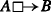, r2 =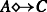, r =C. It can be checked that def({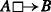C}) = def({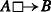,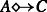}), and so:

$$\mathit{def}(R \cup \{r_{1}, r_{2}, r\}) = \mathit{def}(R \cup \{r_{1}, r_{2}\})$$

This establishes that $$out_{1}(R \cup \{r_{1}, r_{2}, r\},\ A) \subseteq out_{1}(R \cup \{r_{1}, r_{2}\},\ A)$$ for all A. To show the other inclusion, that $$out_{1}(R \cup \{r_{1}, r_{2}\}\ A) \subseteq out_{1}(R \cup \{r_{1}, r_{2}, r\},\ A)$$, it remains to show that if XR ∪{r1, r2} then XR ∪{r1, r2, r}. To see this, note that if Xthen XC. □

### Proposition 6 (Decomposition)

A set Rof rules semantically entails a rule rin KL2if and only if$$R \cup \mathcal {C} \cup aux(R)$$semantically entails rin KL1. That is, for all rule setsR:

$$kl_{2}(R) = kl_{1}(R \cup \mathcal{C} \cup aux(R))$$

### Proof

$$kl_{2}(R) = kl_{1}(R \cup \mathcal {C} \cup aux(R))$$ for all R is equivalent to saying that two rules sets R1 and R2 are strongly equivalent in KL2, kl2(R1) = kl2(R2), iff $$R_{1} \cup \mathcal {C} \cup aux(R_{1})$$ and $$R_{2} \cup \mathcal {C} \cup aux(R_{2})$$ are strongly equivalent in KL1, $$kl_{1}(R_{1} \cup \mathcal {C} \cup aux(R_{1})) = kl_{1}(R_{2} \cup \mathcal {C} \cup aux(R_{2}))$$.

$$\begin{array}{@{}rcl@{}} kl_{2}(R_{1}) &=& kl_{2}(R_{2}) \\ &&\text{iff}\quad out_{2}(R_{1},A) = out_{2}(R_{2},A) \quad \text{for all \textit{A}} \\ &&\text{iff}\quad out_{1}(R_{1} \cup \mathcal{C} \cup aux(R_{1}),\ A) = out_{1}(R_{2} \cup \mathcal{C} \cup aux(R_{2}),\ A) \quad \text{for all \textit{A}} \\ &&\text{iff}\quad kl_{1}(R_{1} \cup \mathcal{C} \cup aux(R_{1})) = kl_{1}(R_{2} \cup \mathcal{C} \cup aux(R_{2})) \end{array}$$

### Proposition 7

Let Rbe a set of rules andAa (finite) set of literals: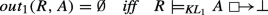### Proof

We need to show that out1(R, A) = iff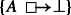for all sets $$A^{\prime }$$ of literals.

For left-to-right: suppose out1(R, A) = . First observe that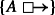for all $$A^{\prime }$$. (Because if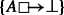then X is computed from assumptions $$A^{\prime }$$ using the non-constraint rules of R and XR. Since XR, that means $$X \in out_{1}(R,A^{\prime })$$ also.)

It remains to show that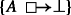for all $$A^{\prime }$$. Assume $$X \in out_{1}(R, A^{\prime })$$; we shall show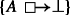. Since $$X \in out_{1}(R, A^{\prime })$$, there is a D in def(R) such that $$X = \mathrm {M}(D, A^{\prime })$$. We will prove, first, that D is one of the definite programs of R, and second, that XR. First, since defr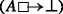= {}, Ddef(R). So D, as well as being one of the definite programs of R, is also one of the definite programs of R. Second, since $$X \in out_{1}(R, A^{\prime })$$, XR. We just need to show X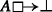. Since out1(R, A) = , $$A \nvDash R$$ by Proposition 2. Now, since XR, $$A \nsubseteq X$$, hence X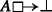. These two claims entail, using Proposition 1, that.

For the other direction: suppose out1(R, A)≠. We need to show that there is some $$A^{\prime }$$ such that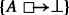. Take $$A^{\prime } = A$$: clearly out1(R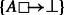, A) = . □

### Proposition 8

Let Rbe a set of rules. If Xis a finite violating set of Rthen: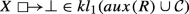### Proof

If X = ( is a violating set of R) then {a} and $$\{{\sim }\mspace {1mu} a\}$$ are also violating sets of R, any atom a, and. Clearly $$aux(R) \cup \mathcal {C}$$ is strongly inconsistent in KL1 and so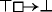(Proposition 4).

Suppose X. If X is a (finite) violating set of R then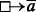. We show that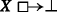by showing that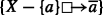.

Consider any rule X −{a}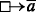, aX. Suppose, for contradiction, that. Then $$X^{\prime } \supseteq X$$ and. In order to satisfy X −{a}, $$X^{\prime }$$ must contain $$\overline {a}$$. But aX so $$X^{\prime }$$ also contains a, and $$X^{\prime } \not \models \mathcal {C}$$. □

### Remark

The proof above shows that if is a violating set of R then $$aux(R) \cup \mathcal {C}$$ is strongly inconsistent in KL1. We can also show that if $$R \cup \mathcal {C}$$ is strongly inconsistent in KL1 then is a violating set of R. (Because then $$out_{1}(R \cup \mathcal {C},X) = \emptyset$$ for every set X of literals including every maximal consistent set X, and is thus a violating set of R.) The converse however is not true. Consider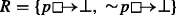. is a violating set of R but R is not strongly inconsistent: out1(R, ) = {}≠.

### Proposition 9

Let Rbe a set of rules and Xa finite set of literals.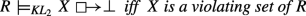### Proof

One half follows from the preceding result: if X is a (finite) violating set of R then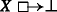; $$kl_{1}(\mathcal {C} \cup aux(R)) \subseteq kl_{1}(R \cup \mathcal {C} \cup aux(R))$$ and so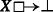kl2(R). It remains to prove that ifkl2(R) then X is a violating set of R. We will prove that if $$out_{1}(R \cup \mathcal {C} \cup aux(R),\ X) = \emptyset$$ then X is a violating set of R.

Consider any definite logic program DR in the encoding def(R) of R. Let def(aux(R)) = {Daux} (all rules in aux(R) are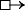rules with singleton heads and so there is a single definite program encoding aux(R)). M(DRDaux, X)⊧aux(R) so it must be that $$\mathrm {M}(D_{R} \cup D_{aux},\ X) \not \models R \cup \mathcal {C}$$, i.e., either M(DRDaux, X) is inconsistent or $$B \subseteq \mathrm {M}(D_{R} \cup D_{aux},\ X)$$ for some rule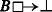in R.

If X is inconsistent then X is a violating set of R. If X is consistent then consider any maximal consistent $$X_{m} \supseteq X$$. $$\mathrm {M}(D_{R} \cup D_{aux},\ X_{m}) \supseteq \mathrm {M}(D_{R} \cup D_{aux},\ X)$$, and since Xm is maximal, $$X_{m} \supseteq \mathrm {M}(D_{R} \cup D_{aux},\ X_{m}) \supseteq \mathrm {M}(D_{R} \cup D_{aux},\ X)$$. If M(DRDaux, X) is inconsistent then so is Xm, and that cannot be. So $$B \subseteq \mathrm {M}(D_{R} \cup D_{aux},\ X)$$ for some rulein R. But then $$B \subseteq X_{m}$$, and XmR. □

### Proposition 11

Let Rbe a set of rules and Aa set of literals.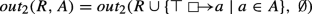### Proof

The result follows from the previous minimality result. It is enough to consider a singleton set of assumptions A = {a}. The general result follows by repeated application.

If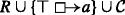is strongly inconsistent the result holds trivially. Suppose it is not strongly inconsistent. We need to show that: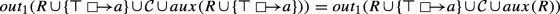We will show that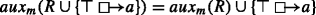.

Clearly $$\{\overline {a}\}$$ and all violating sets of R are violating sets of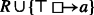. Further, right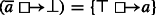. So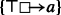. Now (assuming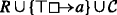is not strongly inconsistent) $$\{\overline {a}\}$$ is a minimal consistent violating set of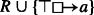. If X is a minimal consistent violating set of R and $$\overline {a} \in X$$ then X is not a minimal consistent violating set of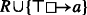; if $$\overline {a} \notin X$$ then X is a minimal consistent violating set of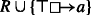. So auxm(R) = auxm(R) ∪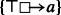. □

### Proposition 15 (Soundness of KL2)

For all setsRof rules:

$$deriv_{2}(R) \subseteq kl_{2}(R)$$

### Proof

We need to show soundness of the inference rules of KL1, $${\sim }\mspace {1mu}$$-LEFT and $${\sim }\mspace {1mu}$$-RIGHT with respect to semantic entailment in KL2. Since $$kl_{2}(R) = kl_{1}(R \cup \mathcal {C} \cup aux(R))$$ (Proposition 6) and KL1 is sound with respect to kl1, the soundness of KL1 inference rules is immediate. Soundness of $${\sim }\mspace {1mu}$$-LEFT is just $$\mathcal {C} \subseteq kl_{2}(R)$$ which also follows trivially.

It remains to show that $${\sim }\mspace {1mu}$$-RIGHT is sound: if rright(R) then $$R \models _{\text {KL}_{2}} r$$, or more generally, that $$right(R) \subseteq kl_{2}(R)$$. We want to show:

$$right(R) \subseteq kl_{1}(R \cup \mathcal{C} \cup aux(R))$$

We will show that $$right(R) \subseteq aux(R)$$ (which implies the above). In full: if rright(R) then r is a rule of the form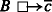where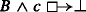is a rule in R. In that case B ∪{c} is a (not necessarily minimal or consistent) violating set of R, and aux(R) contains the rule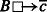. □

### Proposition 16 (Conditional completeness of KL2)

If KL1is complete with respect to out1then KL2is complete with respect to out2. That is: if, for all sets Rof rules$$kl_{1}(R) \subseteq deriv_{1}(R)$$then, for all sets Rof rules$$kl_{2}(R) \subseteq deriv_{2}(R)$$.

### Proof

$$\begin{array}{@{}rcl@{}} r \in kl_{2}(R) \ &\Rightarrow&\ r \in kl_{1}(R \cup \mathcal{C} \cup aux(R)) \\ &\Rightarrow&\ r \in kl_{1}(R \cup \mathcal{C} \cup aux_{e}(R)) \quad\qquad\qquad\qquad\qquad\hfill(\text{Proposition~13}) \\ &\Rightarrow&\ r \in deriv_{1}(R \cup \mathcal{C} \cup aux_{e}(R)) \qquad\qquad\quad\hfil(\text{completeness of KL}_{1}) \\ &\Rightarrow&\ r \in deriv_{2}(R) \end{array}$$

The final step is because all the inference rules used in the construction of auxe(R) in Proposition 14 are inference rules of KL2. □

### Proposition 17 (Decomposition of KL2)

If KL 1 is complete with respect to o u t 1 then

$$deriv_{2}(R) = deriv_{1}(R \cup \mathcal{C} \cup aux_{e}(R))$$

### Proof

Right-in-left inclusion is noted in the proof of Proposition 16. The other inclusion is similar:

$$\begin{array}{@{}rcl@{}} r \in deriv_{2}(R) \ &\Rightarrow&\ r \in kl_{2}(R)\qquad\qquad\qquad\qquad\qquad\qquad \quad\hfil(\text{soundness of KL}_{2}) \\ &\Rightarrow&\ r \in kl_{1}(R \cup \mathcal{C} \cup aux(R)) \\ &\Rightarrow&\ r \in kl_{1}(R \cup \mathcal{C} \cup aux_{e}(R))\qquad\qquad\qquad\qquad \hfil(\text{Proposition~13}) \\ &\Rightarrow&\ r \in deriv_{1}(R \cup \mathcal{C} \cup aux(R))\qquad\qquad \hfil(\text{completeness of KL}_{1}) \end{array}$$

### Proposition 19

LetRbe a set of rules and Aa set of assumptions, both containing no negative literals. Then:

$$out_{2}(R,A)^{+} = out_{1}(R,A)$$

### Proof

We can see this again by looking at the rules in aux(R): if R contains no negative literals, all rules in aux(R) are of the form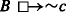where c and all of B are atoms. These rules have no effect on the outcomes computed from R except possibly to add negative literals. This is clear if we look at the translation to definite logic programs: every definite program D in the encoding $$\mathit {def}(R \cup \mathcal {C} \cup aux(R))$$ has the form DRDaux where DRdef(R) and def(aux(R)) = {Daux} (all rules in aux(R) arerules with singleton heads and so there is a single definite program encoding aux(R)). Moreover, since none of the heads of clauses in Daux appear in DR the least model M(DRDaux, A) = M(DR,M(Daux, A)) (indeed that is true whether the set A of assumptions contains negative literals or not). If A contains no negative literals, then this least model also satisfies the constraints $$\mathcal {C}$$. □